• 前言 在之前的这篇文章里，笔者详细讲解了二叉堆这种简单有效的数据结构，并且讨论了优先队列的实现方式。现在有个新的问题： 给定两个优先队列，请尽量高效地将它们合并成一个优先队列。...左偏树（leftist tree）...
前言
在之前的这篇文章里，笔者详细讲解了二叉堆这种简单有效的数据结构，并且讨论了优先队列的实现方式。现在有个新的问题：

给定两个优先队列，请尽量高效地将它们合并成一个优先队列。

很显然，如果我们使用二叉堆实现，只能先出队再入队，而线性复杂度显然不能令人满意。所以我们需要考虑可合并堆（mergeable heap），常见的可合并堆有如下四种：
左偏树（leftist tree）二项堆（binomial heap）斐波那契堆（Fibonacci heap）配对堆（pairing heap）
其中前两者可以实现O(logn)的合并，而后两者更是可以达到O(1)。限于篇幅，本文只讨论左偏树，因为它是可合并堆中最简单的一种，容易编码实现，“性价比”比较高，应用也较广泛。
左偏树
定义与性质
顾名思义，左偏树就是一棵往左偏的树（废话
左偏树是一棵二叉树，但是它的每个节点上除了记录键值之外，还会记录距离值（dist值，又称npl或者s-value）。所谓距离值，就是指树中某个节点与其距离最近的外节点之间的路径长度。而外节点（external node）就是那些没有子节点或者只有1个子节点的节点，特别地，规定外节点的距离值为0，而NULL节点的距离值为-1。
下图来自百度百科，是一个用左偏树表示的最小堆，蓝色数字就是距离值。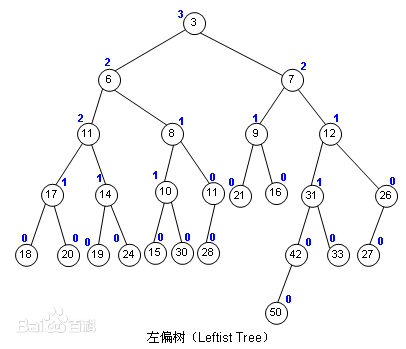以下都以最小堆为例，左偏树具有以下两个性质：

堆性质：每个节点存储的值都小于或等于其子节点存储的值。 左偏性质：每个节点的左子节点的距离值总是不小于右子节点的距离值。

看官可以再观察一下上面的图，以方便理解这两个性质。可见，左偏树的子树也都是左偏树。
注意：左偏树并不一定像二叉堆那样是一棵完全二叉树。并且判断左偏的标准是距离值，所以左子树的深度或者节点数未必大于右子树。
根据左偏性质和距离值的定义，容易得出推论：

左偏树中一个节点的距离值等于它的右子节点距离值加上1。

另外再根据二叉树的性质，容易得出以下推论：

如果一棵左偏树根节点的距离值为d，那么该左偏树最少可以有2d+1-1个节点，此时它才是完全二叉树。 换言之，一棵有n个节点的左偏树，其根节点的距离值最大为⌊log(n+1)⌋-1。

接下来具体看看左偏树最重要的合并操作的流程。
合并操作
合并两棵左偏树A和B之前，先检查它们两个的根节点的值，较小的那个作为合并结果树的根节点。现假设A的根节点较小，那么我们把A的左子树放置不动，把A的右子树递归地与B进行合并。递归返回时，需要更新结果树的根节点距离值。另外，如果结果树不满足左偏性质，就将其左右子树交换，以使其再度满足左偏性质。简单的示意图如下所示。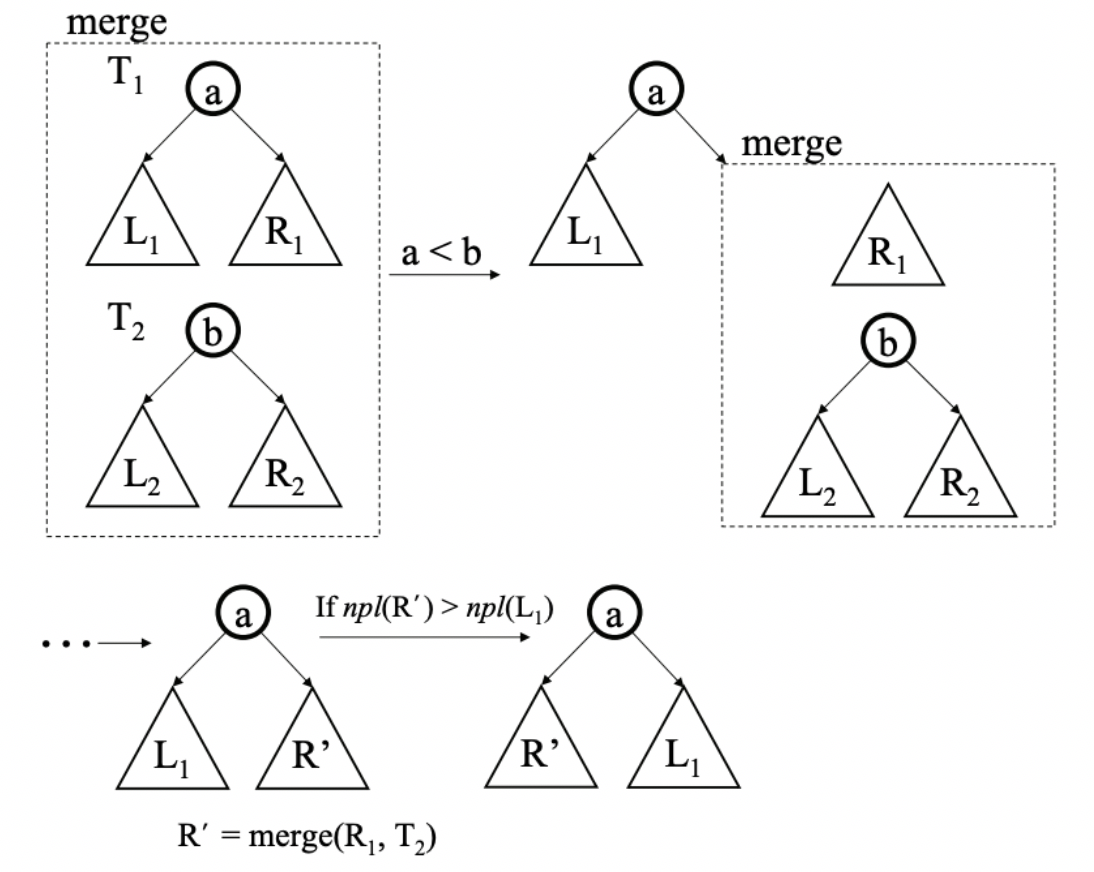上图可能不太直观，接下来举一个更实际一些的例子。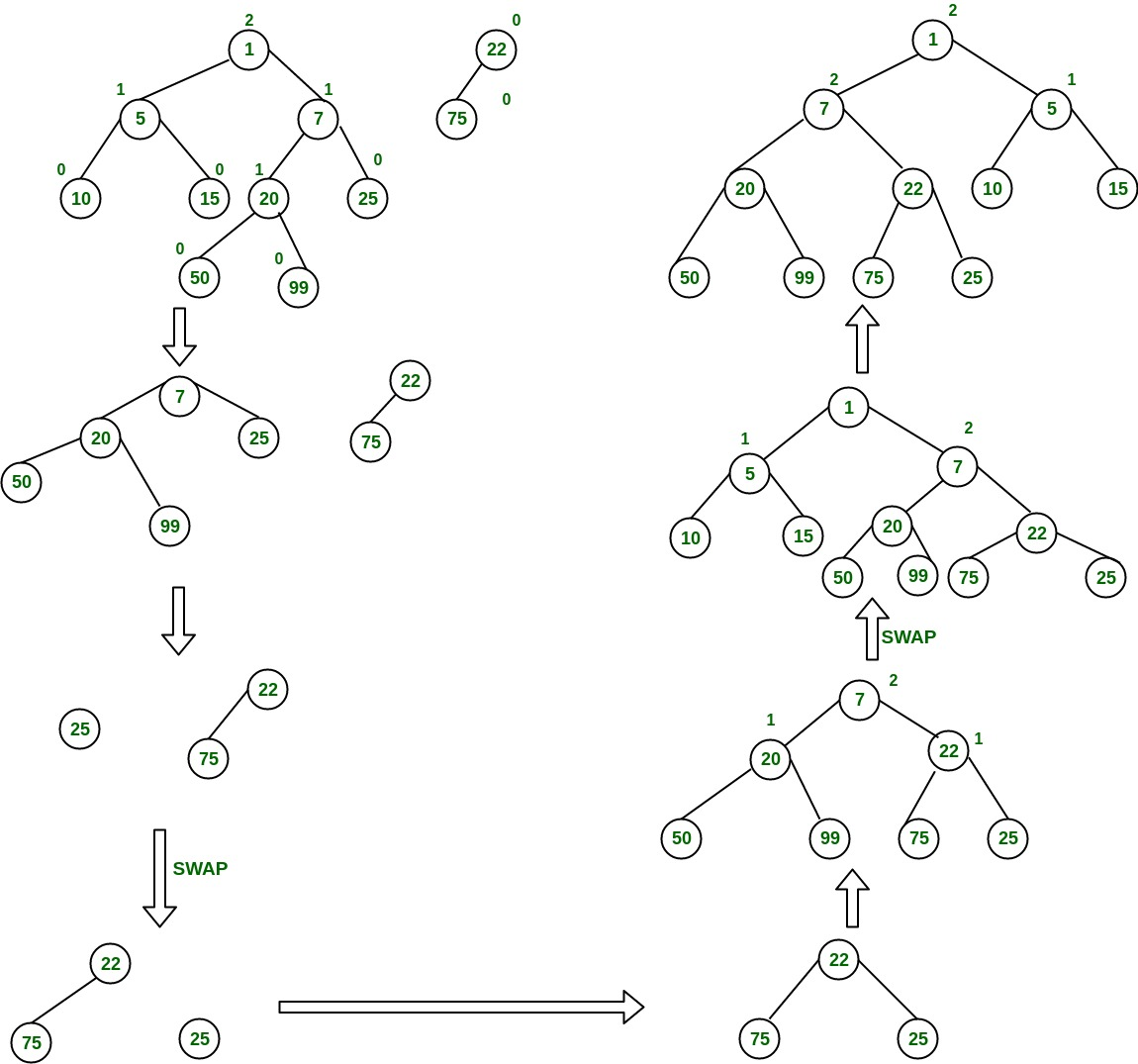由此可见，左偏性质使得我们每次都可以只在右子树上进行合并操作。最后用伪码来表达其流程如下。
function merge(a, b) {
if (a == null)
return b;
if (b == null)
return a;
if (a.val > b.val)
swap(a, b);

a.right = merge(a.right, b);

if (a.left.dist < a.right.dist)
swap(a.left, a.right);

if (a.right == null)
a.dist = 0;
else
a.dist = a.right.dist + 1;

return a;
}

整个合并操作的实现是很简洁的。根据上一节的推论，可以得出合并操作的最坏时间复杂度为O(log|A|+log|B|)。
插入&构造操作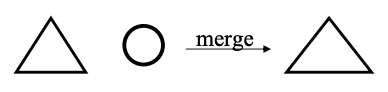有了合并操作做基础，插入操作实际上就是合并一棵已有的左偏树和一棵单节点的左偏树，即：
function insert(a, x) {
b = make_leftist_tree(x);
return merge(a, b);
}

显然，如果我们用插入操作从零开始构造n个节点的左偏树，时间复杂度为O(nlogn)。一种优化方法是采用普通的FIFO队列来辅助构造，每次从队头取出两棵左偏树合并后放入队尾，直到队列中只剩一棵树为止，可以达到O(n)。
取堆顶操作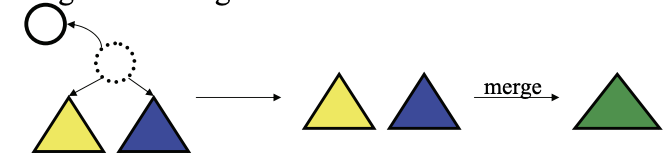取堆顶也就是弹出左偏树中的最小元素。将其根节点移除后，剩下的两棵左右子树也都是左偏树，再把它们合并到一起就OK了。
function pop(a) {
v = a.val;
a = merge(a.left, a.right);
return v;
}

说了这么多，看一道简单的例题吧。
例题：HDOJ 1512
传送门在这里。题目大意：有N只猴子，每只猴子起初互相都不认识。猴子之间经常发生冲突，每次会有两只猴子（a和b）约架，并且a和b各自会邀请自己的朋友中战斗力最强的那只（包括它自己）上场。每打完一场后，上场的两只猴子战斗力减半，并且它们和它们的朋友都会互相成为朋友，成为朋友的猴子就不会再约架。输入猴子总数、战斗力值和每次约架的猴子编号，要求输出每次打完后最高的战斗力值。
显然，判断是否为朋友可以用并查集实现（之前已经讲过），找最大值可以用最大堆实现。但是并查集每次合并之后，堆也要随着更新，普通二叉堆自然是不行的，左偏树就是解决这种问题的利器了。
直接放出AC代码如下。注意并查集的合并和左偏树的合并逻辑一起放在了merge()方法中。
#include <iostream>
#include <cstdio>
#include <algorithm>
using namespace std;

const int MAXN = 100002;

struct LTNode {
int left, right, dist, str;
}ltree[MAXN];

int parent[MAXN];
int n, m, a, b;

int find(int x) {
int r = x;
while (parent[r] != r) {
r = parent[r];
}
int p = parent[x];
while (p != r) {
parent[x] = r;
x = p;
p = parent[x];
}
return r;
}

int merge(int x, int y) {
if (x == 0) {
return y;
}
if (y == 0) {
return x;
}
if (ltree[x].str < ltree[y].str) {
swap(x, y);
}
ltree[x].right = merge(ltree[x].right, y);
parent[ltree[x].right] = x;
if (ltree[ltree[x].left].dist < ltree[ltree[x].right].dist) {
swap(ltree[x].left, ltree[x].right);
}
ltree[x].dist = ltree[x].right == 0 ? 0 : ltree[ltree[x].right].dist + 1;
return x;
}

int pop(int x) {
int l = ltree[x].left, r = ltree[x].right;
parent[l] = l;
parent[r] = r;
ltree[x].left = ltree[x].right = ltree[x].dist = 0;
return merge(l, r);
}

int main() {
while (~scanf("%d", &n)) {
memset(ltree, 0, sizeof(ltree));
for (int i = 1; i <= n; i++) {
scanf("%d", &ltree[i].str);
parent[i] = i;
}
scanf("%d", &m);
while (m--) {
scanf("%d%d", &a, &b);
int ra = find(a), rb = find(b);
if (ra == rb) {
printf("-1\n");
continue;
} else {
int pa = pop(ra), pb = pop(rb);
ltree[ra].str /= 2;
ltree[rb].str /= 2;
pa = merge(pa, ra);
pb = merge(pb, rb);
printf("%d\n", ltree[merge(pa, pb)].str);
}
}
}
return 0;
}

民那晚安。
展开全文• 开两个左偏树：H1H_1H1​维护NNN个节点，H2H_2H2​维护H1H_1H1​堆顶元素 UUU：H1H_1H1​直接连边，H2H_2H2​删去一个点 （先判断是否在同一堆中） A1A_1A1​：H1H_1H1​—将xxx点取出来，加上vvv后...
解析

P

a

r

t

0

Part_0

：吐槽 我错了，这种毒瘤题真的不适合我这种退役狗 表示被负数坑死了

P

a

r

t

1

Part_1

：思路 开两个左偏树：

H

1

H_1

维护

N

N

个节点，

H

2

H_2

维护

H

1

H_1

堆顶元素

U

U

：

H

1

H_1

直接连边，

H

2

H_2

删去一个点 （先判断是否在同一堆中）

A

1

A_1

：

H

1

H_1

—将

x

x

点取出来，加上

v

v

后再合并上去 （要加上

l

a

z

y

lazy

未下传的值）

H

2

H_2

—将

H

1

H_1

的原堆顶取出，再将新堆顶合并

A

2

A_2

：

H

1

H_1

打标记，

H

2

H_2

同于

A

1

A_1

中

H

1

H_1

的操作

A

3

A_3

：直接一个变量记录即可

F

1

F_1

：直接在

H

1

H_1

中查询（同上要加上

l

a

z

y

lazy

的值）

F

2

F_2

：

H

1

H_1

堆顶

F

3

F_3

：

H

2

H_2

堆顶 PS：貌似可以用并查集优化，但是已经码得心累了，

G

i

v

e

Give

U

p

Up

P

a

r

t

2

Part_2

：左偏树涉及操作 初始化、合并、删除（已知节点）

代码
#include <cstdio>
#include <cstdlib>
#include <cstring>
#include <algorithm>
#include <queue>

#define IL inline

using namespace std;

{
int sum = 0;
bool f = 0;
char c = getchar();
for(; '0' > c || c > '9'; c = getchar())
if(c == '-') f = 1;
for(; '0' <= c && c <= '9'; c = getchar())
sum = sum * 10 + (c - '0');
return f ? -sum : sum;
}

const int N(300005);

queue<int>Q;

struct zps
{
int ch[N], pa[N], val[N], dis[N], lazy[N];
IL int Get_val(int x)
{
int s = val[x];
for(x = pa[x]; x; x = pa[x]) s += lazy[x];
return s;
}

IL int Find(int x)
{
for(; pa[x]; x = pa[x]);
return x;
}

IL void Clean(int x)
{
if(!lazy[x]) return ;
int y;
if((y = ch[x])) { val[y] += lazy[x]; lazy[y] += lazy[x]; }
if((y = ch[x])) { val[y] += lazy[x]; lazy[y] += lazy[x]; }
lazy[x] = 0;
}

IL int Merge(int x, int y)
{
if(!x) return y;
if(!y) return x;
if(val[x] < val[y]) swap(x, y);
Clean(x);
ch[x] = Merge(ch[x], y);
pa[ch[x]] = x;
if(dis[ch[x]] < dis[ch[x]]) swap(ch[x], ch[x]);

dis[x] = dis[ch[x]] + 1;

return x;
}

IL void Clear(int x)
{
pa[x] = ch[x] = ch[x] = 0;
}

IL int Delete(int x)
{
Clean(x);
int tmp = Merge(ch[x], ch[x]), p = pa[x];
pa[tmp] = p;
if(p) ch[p][(x == ch[p])] = tmp;
Clear(x);
for(; p;)
{
if(dis[ch[p]] < dis[ch[p]]) swap(ch[p], ch[p]);
if(dis[p] == dis[ch[p]] + 1) return Find(p);
dis[p] = dis[ch[p]] + 1;
tmp = p;
p = pa[p];
}
return tmp;
}

IL int Add(int x, int v)
{
val[x] += v;

//没什么用的优化
Clean(pa[x]); Clean(x);
if(val[pa[x]] >= val[x] && val[x] >= val[ch[x]] && val[x] >= val[ch[x]]) return Find(x);

val[x] = Get_val(x);
return Merge(Delete(x), x);
}

IL int build()
{
for(int t = Q.size(), p1, p2; t > 1; --t)
{
p1 = Q.front(); Q.pop();
p2 = Q.front(); Q.pop();
Q.push(Merge(p1, p2));
}
return Q.front();
}

}h1, h2;

int main()
{

int root2 = 0;

for(int i = 1; i <= n; ++i)
{
Q.push(i);
}

root2 = h2.build();

char op = {0};
for(int m = read(), x, y, tmp, tmp2; m; --m)
{
scanf(" %s", op);
if(op == 'U')
{
if(x == y) continue;
root2 = h2.Delete((h1.Merge(x, y) == x ? y : x));
}else
if(op == 'A' && op == '1')
{
tmp = h1.Find(x);

/*root2 = h2.Delete(tmp);
h2.val[tmp] = h1.val[tmp];
root2 = h2.Merge(root2, tmp);*/
//没什么用的优化
if(tmp ^ tmp2)
{
root2 = h2.Delete(tmp);
h2.val[tmp2] = h1.val[tmp2];
root2 = h2.Merge(root2, tmp2);
}else
if(x == tmp)
{
}

}else
if(op == 'A' && op == '2')
{
h1.val[x] += y; h1.lazy[x] += y;
}else
if(op == 'A' && op == '3')
{
}else
if(op == 'F' && op == '1')
{
printf("%d\n", tmp);
}else
if(op == 'F' && op == '2')
{
}else
if(op == 'F' && op == '3')
{
}
}

return 0;
}

展开全文• 左偏树是一种二叉堆，这种堆可以合并出高度比较低的树，具体就是通过节点x到没有右子树的节点y的距离来进行判断，及时交换左右孩子，从而使得堆不会变得很长。 这样的堆有利于再和其他的堆进行合并合并的时候...

Description 在一个忍者的帮派里，一些忍者们被选中派遣给顾客，然后依据自己的工作获取报偿。在这个帮派里，有一名忍者被称之为 Master。除了 Master以外，每名忍者都有且仅有一个上级。为保密，同时增强忍者们的领导力，所有与他们工作相关的指令总是由上级发送给他的直接下属，而不允许通过其他的方式发送。现在你要招募一批忍者，并把它们派遣给顾客。你需要为每个被派遣的忍者 支付一定的薪水，同时使得支付的薪水总额不超过你的预算。另外，为了发送指令，你需要选择一名忍者作为管理者，要求这个管理者可以向所有被派遣的忍者 发送指令，在发送指令时，任何忍者（不管是否被派遣）都可以作为消息的传递 人。管理者自己可以被派遣，也可以不被派遣。当然，如果管理者没有被排遣，就不需要支付管理者的薪水。你的目标是在预算内使顾客的满意度最大。这里定义顾客的满意度为派遣的忍者总数乘以管理者的领导力水平，其中每个忍者的领导力水平也是一定的。写一个程序，给定每一个忍者 i的上级 Bi，薪水Ci，领导力L i，以及支付给忍者们的薪水总预算 M，输出在预算内满足上述要求时顾客满意度的最大值。

1 ≤N ≤ 100,000 忍者的个数； 1 ≤M ≤ 1,000,000,000 薪水总预算； 0 ≤Bi < i 忍者的上级的编号； 1 ≤Ci ≤ M 忍者的薪水； 1 ≤Li ≤ 1,000,000,000 忍者的领导力水平。

Input 从标准输入读入数据。 第一行包含两个整数 N和 M，其中 N表示忍者的个数，M表示薪水的总预算。 接下来 N行描述忍者们的上级、薪水以及领导力。其中的第 i 行包含三个整 Bi , C i , L i分别表示第i个忍者的上级，薪水以及领导力。Master满足B i = 0，并且每一个忍者的老板的编号一定小于自己的编号 Bi < i。 Output 输出一个数，表示在预算内顾客的满意度的最大值。

Sample Input 5 4 0 3 3 1 3 5 2 2 2 1 2 4 2 3 1 Sample Output 6

HINT 如果我们选择编号为 1的忍者作为管理者并且派遣第三个和第四个忍者，薪水总和为 4，没有超过总预算 4。因为派遣了 2 个忍者并且管理者的领导力为3，用户的满意度为 2,是可以得到的用户满意度的最大值。

我们需要处理出每个节点的孩子中满足条件的尽可能多的节点，但是我们如果从上往下遍历处理的话显然会超时O(n^2)。
所以我们需要从下往上进行处理，尽可能地利用以前的信息，所以不妨对每个节点使用一个优先队列，将满足条件的都放进去，如果工资已经高于能负担的工资，就把工资最高的那个人去掉。然后将相邻两个节点进行合并。为了提高效率，我们应当先处理重孩子，再处理轻孩子。
可是这样使用STL自带的优先队列复杂度还不够优秀，我们可以针对这个问题实现我们自己的优先队列，所用的数据结构就是左偏树
左偏树是一种二叉堆，这种堆可以合并出高度比较低的树，具体就是通过节点x到没有右子树的节点y的距离来进行判断，及时交换左右孩子，从而使得堆不会变得很长。
这样的堆有利于再和其他的堆进行合并。
合并的时候：从上往下合并，不断地把根节点更大的右儿子和另一个堆合并。（可以证明这样的复杂度最优秀）
具体到这个问题中，我们用节点类型存储每个节点的数据，可是每个节点的根节点却不一定是他本身，在二叉堆构造过程中，原本的位置已经改变，为了记录这种改变，我们用root数组记录在左偏树中该节点的父亲节点。（在访问到他为止左偏树中的节点都是实际树形关系中他的孩子和他本身）
当目前工资已经超过最大工资的时候，我们就删去堆顶元素（贪心思想，堆顶的工资最高，删去他最划算）
借鉴了网上的代码加上自己的思考，发现自己这样写虽然没有什么错误，但是还是应该把用于记录堆关系的和用于记录树关系的数据分开，混在一起导致容易理解错误。
#include<iostream>
#include<cstring>
#include<cstdio>
#include<climits>
#include<algorithm>
#include<ctime>
#include<cstdlib>
#include<queue>
#include<set>
#include<map>
#include<cmath>
#define rep(i,x,n) for(register int i=x;i<=n;i++)

using namespace std;

typedef long long ll;
const int MAXN=1e5+5;
struct Node
{
int cost; ll ctrl;
int rson,lson,dis;
}Tree[MAXN];
int nex[MAXN];
int N; ll M;
int root[MAXN],sz[MAXN]; ll sum[MAXN];
ll ans=0;

int Merge(int x,int y)
{
if(!x || !y) return x+y;
if(Tree[x].cost<Tree[y].cost) swap(x,y);
Tree[x].rson=Merge(Tree[x].rson,y);
if(Tree[Tree[x].rson].dis> Tree[Tree[x].lson].dis) swap(Tree[x].rson,Tree[x].lson);
Tree[x].dis=Tree[Tree[x].rson].dis+1;
return x;
}

void Delete(int& x)
{
x=Merge(Tree[x].lson,Tree[x].rson);
}

int dfs(int x)
{
root[x]=x;
sum[x]=Tree[x].cost; sz[x]=1;
{
dfs(i);
root[x]=Merge(root[x],root[i]);
sum[x]+=sum[i]; sz[x]+=sz[i];
}
while(sum[x]>M)
{
sum[x]-=Tree[root[x]].cost;
sz[x]--;
Delete(root[x]);
}
ans=max(ans,sz[x]*Tree[x].ctrl);
}

int main()
{
scanf("%d%lld",&N,&M);
int t; //int Master=-1;
rep(i,1,N)
{
scanf("%d",&t);
//if(t==0) Master=i;
scanf("%d%lld",&Tree[i].cost,&Tree[i].ctrl);
}
dfs(1);
printf("%lld",ans);
return 0;
}


展开全文• 【算法简介】 顾名思义，这个数据结构向左偏，而且主要的性质是可以合并，而且是一个有序的堆 ...还有一个删除操作，左偏树不支持删除某个值得点的操作，只能删除某个已知位置的点，一般常用的操作是删除根节点 【例
【算法简介】
顾名思义，这个数据结构向左偏，而且主要的性质是可以合并，而且是一个有序的堆
定义外节点是有一个儿子是空的的节点，每个点的dis定义为到最近的外节点经过的边的数量
我们让每个节点的左儿子的dis>=右儿子的dis，然后操作尽量从右侧开始，通过这种方式来保证其优秀的时间效率
合并操作就是每次让右侧儿子和另一棵树进行合并，合并的过程中注意满足有序的性质，还有左偏的性质就行
还有一个删除操作，左偏树不支持删除某个值得点的操作，只能删除某个已知位置的点，一般常用的操作是删除根节点
【例题】P1552 [APIO2012]派遣
【题意】
【分析】
【代码】
#include<bits/stdc++.h>
using namespace std;
const int maxn=1e5+5;
typedef long long ll;
int n,m;
ll c[maxn],l[maxn];
struct edge
{
int to,nxt;
}e[maxn];
{
}
int root,b;
struct tree
{
int ls,rs,fa;
ll val,dis;
}tr[maxn];
ll siz[maxn],valu[maxn];
int merge(int x,int y)
{
if(!x || !y) return x+y;
if(tr[x].val<tr[y].val) swap(x,y);
tr[x].rs=merge(tr[x].rs,y);
if(tr[tr[x].rs].dis>tr[tr[x].ls].dis) swap(tr[x].ls,tr[x].rs);
if(!tr[x].rs || !tr[x].ls) tr[x].dis=0;
else tr[x].dis=tr[tr[x].rs].dis+1;
return x;
}
ll ans;
int del(int x)
{
int l=tr[x].ls,r=tr[x].rs;
tr[l].fa=l; tr[r].fa=r;
tr[x].ls=tr[x].rs=tr[x].dis=0;
return merge(l,r);
}
int id[maxn];
void dfs(int u)
{
siz[u]=1; valu[u]=c[u];
{
int to=e[i].to;
dfs(to);
id[u]=merge(id[u],id[to]);
siz[u]+=siz[to]; valu[u]+=valu[to];
}
while(valu[u]>m)
{
siz[u]--; valu[u]-=tr[id[u]].val;
id[u]=del(id[u]);
}
ans=max(ans,l[u]*siz[u]);
}
int main()
{
freopen("dispatching.in","r",stdin);
freopen("dispatching.out","w",stdout);
scanf("%d%d",&n,&m);
for(int i=1;i<=n;i++)
{
scanf("%d%d%d",&b,&c[i],&l[i]);
if(!b) root=i;
else
id[i]=i;
}
tr.dis=-1;
for(int i=1;i<=n;i++) tr[i].fa=i,tr[i].val=c[i];
dfs(root);
printf("%lld\n",ans);
return 0;
}

【练习1】P3261 [JLOI2015]城池攻占
【题意】
题意好麻烦
【分析】
开始把士兵加到各个节点的队列中，类似例题，做dfs的过程中，合并堆，每次删除hp<0的士兵，并记录death和num的贡献
每个节点的修改可以记录在标记上，注意及时下传即可
【代码】
#include<bits/stdc++.h>
using namespace std;
const int maxn=3e5+5;
typedef long long ll;
int n,m;
int s[maxn],dep[maxn],fa[maxn];
ll num[maxn],death[maxn],h[maxn];
struct edge
{
int to,nxt;
}e[maxn];
struct tree
{
int ls,rs,fa;
ll val,dis,mtag,atag;
}tr[maxn];
{
}
int root[maxn];
void pushdown(int x)
{
ll mt=tr[x].mtag,at=tr[x].atag;
if(tr[x].ls)
{
tr[tr[x].ls].mtag*=mt; tr[tr[x].ls].atag*=mt;
tr[tr[x].ls].atag+=at; tr[tr[x].ls].val=tr[tr[x].ls].val*mt+at;
}
if(tr[x].rs)
{
tr[tr[x].rs].mtag*=mt; tr[tr[x].rs].atag*=mt;
tr[tr[x].rs].atag+=at; tr[tr[x].rs].val=tr[tr[x].rs].val*mt+at;
}
tr[x].mtag=1; tr[x].atag=0;
}
int merge(int x,int y)
{
if(!x || !y) return x+y;
pushdown(x),pushdown(y);
if(tr[x].val>tr[y].val) swap(x,y);
tr[x].rs=merge(tr[x].rs,y);
if(tr[tr[x].ls].dis<tr[tr[x].rs].dis) swap(tr[x].ls,tr[x].rs);
tr[x].dis=tr[tr[x].rs].dis+1;
return x;
}
void dfs(int u)
{
dep[u]=dep[fa[u]]+1;
{
int to=e[i].to;
dfs(to);
root[u]=merge(root[u],root[to]);
}
while(tr[root[u]].val<h[u] && root[u])
{
pushdown(root[u]);
death[u]++;
num[root[u]]=dep[c[root[u]]]-dep[u];
root[u]=merge(tr[root[u]].ls,tr[root[u]].rs);
}
if(!op[u])
{
tr[root[u]].atag+=v[u];
tr[root[u]].val+=v[u];
}
else
{
tr[root[u]].mtag*=v[u];
tr[root[u]].atag*=v[u];
tr[root[u]].val*=v[u];
}
}
int main()
{
freopen("city.in","r",stdin);
freopen("city.out","w",stdout);
scanf("%d%d",&n,&m);
for(int i=1;i<=n;i++) scanf("%lld",&h[i]);
int x;
for(int i=2;i<=n;i++)
{
scanf("%d%d%lld",&fa[i],&op[i],&v[i]);
}
for(int i=1;i<=m;i++)
{
scanf("%lld%d",&tr[i].val,&c[i]);
root[c[i]]=merge(root[c[i]],i);
tr[i].mtag=1;
}
dfs(1);
while(root)
{
pushdown(root);
num[root]=dep[c[root]];
root=merge(tr[root].ls,tr[root].rs);
}
for(int i=1;i<=n;i++) printf("%lld\n",death[i]);
for(int i=1;i<=m;i++) printf("%lld\n",num[i]);
return 0;
}


展开全文• 左偏树是可合并的二叉堆，首先满足所有的堆的性质，其外，它还可以合并左偏树的树节点需要保存的信息有：  1.左右子树节点编号  2.此节点到有空子结点的结点的最短距离len  3.自身权值 首先解释一下什么是有...
• 1、左偏树 1.1定义 左偏数是一颗二叉树，并具有堆性质。左偏树具有两个属性：键值(key)和距离(dist)。 键值(key)：用于节点比较大小的属性，类似于堆中节点的键值 外节点：左子树或右子树为空的节点称为外节点，...数据结构 二叉树
• 随机生成两棵树的节点，按照插入节点的方法形成两棵左偏树，分别以层次遍历的方法输出这两棵树，然后按照合并算法，再输出合并后的左偏树
• 左偏树习题 1.板题 P3377 https://harris.blog.csdn.net/article/details/121011654 (参考私密) 2.P2713 板题的双倍经验。 // Problem: P2713 罗马游戏 // Contest: Luogu // URL: ...
• 于是很想码左偏树，发现竟然没有水左偏树的blog，所以来一发不要建议。 看到CODE[VS]有道合并果子++，数据大了100倍，那只能用单调序列了。#include #include using namespace std; const int ma
• 左偏树(可合并堆)： 有时需要将两个堆合并，此时每次都对一个pop()再在另一个push() ，太慢了。此时直接合并堆会更快，设堆1有size1个节点，堆2有size2个节点，则合并堆1堆2的复杂度为：O(log(size1))+O(log(size2))...算法
• ## 算法详解——左偏树

万次阅读 多人点赞 2018-06-07 21:59:25
可并堆是啥 ...这个时候，就要用一种神奇的数据结构：左偏树了。 （二项堆，斐波那契堆不在本文的考虑范围内） 左偏树 顾名思义，左偏树就是一棵向左偏的树（逃 我们定义一个...
• 黄源河的左偏树论文，一种简单易写而且合并和取最小值复杂度很低的平衡树
• //本文转自：这里 ... Minimum, Delete-Min)，还支持一个额外的操作——合并操作： H ← Merge(H1,H2) Merge( ) 构造并返回一个包含H1和H2所有元素的新堆H。   O(n)，用它来实现可
• 一、左偏树的性质 左偏树，又称可并堆，所以他有堆的性质。 定义几个量：$$val$$表示该节点的值，$$fa$$表示该节点的父亲，$$ch$$表示该节点的两个儿子（因为他是二叉树）,$$dis$$表示这个节点到离他最近的叶子...
• 左偏树是一种优先队列,它除了支持优先队列的三个操作:插入,取得最小(最大)节点,删除最小(最大)节点之外,还支持一个额外的操作:合并操作 左偏树是一种可并堆,它以一棵二叉树的形式存在.二叉树中每一个节点保存有左右...
• 今天介绍另一款重量级嘉宾：基于左偏树的可并堆(Mergeable Heap)。从应用层面来讲，它完全兼容二叉堆的操作，而且另外添加了一个扩展功能：将两个堆合并起来。可并堆的优势在于：如果将两个普通的沙堆进行合并，那么...数据结构 抽象代数 算法 队列
• ## 左偏树（可并堆）

千次阅读 2018-08-17 19:06:00
左偏树其实是一种可并堆，它可以 O(log2n)O(log2n)O(log_2 n) 合并两个堆。 那左偏？也就是说他左边肯定有什么东西比右边大…… 别着急，在左偏树上有一个叫距离的东西： 个点的距离，被定义为它子树中离他最近的...
• 这一周的任务就是熟悉一下...可并堆，顾名思义就是可以合并的堆 我们一般概念上的堆就是一种优先队列 可是如果要求把两个堆合并，要怎么办呢？ 这时我们就需要一种新的数据结构： 可并堆(Mergeable Heap) ...
• 左偏树相对于二叉堆，其插入，弹出，合并的时间复杂度都是对数级别的。 高度优先左偏树（HBLT） 考虑一颗二叉树，将其叶子节点的子节点补全，那么原来有的节点叫做内部节点，新增加的节点叫做外部节点。 令s(x)s(x)s...
• //这个根据所须的左偏树是大根堆还是小根堆，这里是小根堆，反过来是大根堆 rs ( x ) = merge ( rs ( x ) , y ) ; //将右子树与y合并 f [ rs ( x ) ] = x ; //当需要更新父亲时加上 t [ x ] . d = t [ rs ( x...
• 它满足堆的性质，并且能在log的时间复杂度下合并两棵左偏树左偏树的定义： 定义1左偏树中的一个节点，如果它的右子树为空，则称它是一个外结点。 定义2对于左偏树中的一个节点x，到它的子节点中，离它最近的...
• 最近学了左偏树，学的时候深感网上没有详细教程之苦，所以自己来写一篇（因为是蒟蒻所以可能写的不是很好） 左偏树是什么？ 左偏，顾名思义，就是往左倾斜，左偏树既满足堆的性质，又满足左偏的性质，实质上，左偏...
• 左偏树(Leftist Tree) 是一种可并堆(Mergeable Heap) ，它除了支持优先队列的三个基本操作（插入，删除，取最小节点），还支持一个很特殊的操作——合并操作。 左偏树是一棵堆有序(Heap Ordered)二叉树。 左偏树...
• [可并堆与左偏树] ...左偏树是一种可并堆(MergeableHeap)，意思是可以在O(logN)时间内完成两个堆的合并操作。左偏树(LeftistTree)，或者叫左倾树，左式树，左式堆(LeftistHeap)，左堆。顾名思义，它...
• 左偏树 定义 左偏树(leftist tree 或 leftist heap)，又被成为左倾堆、左偏堆，最左堆等。 左倾树是一棵二叉树，它的节点除了和二叉树的节点一样具有左右子树指针外，还有两个属性：键值和零距离。(01) 键值的作用是...二项树 二项堆 斐波那契堆
• Part 1 左偏树是干嘛的首先，它支持的是两个堆的合并过程。那么最容易想到的是把一个堆的元素全部弹出，一个一个加入另一个堆中，就合并了。显然这样合并的复杂度是O(n)的，再加上程序的其他部分，很慢。我们就考虑......